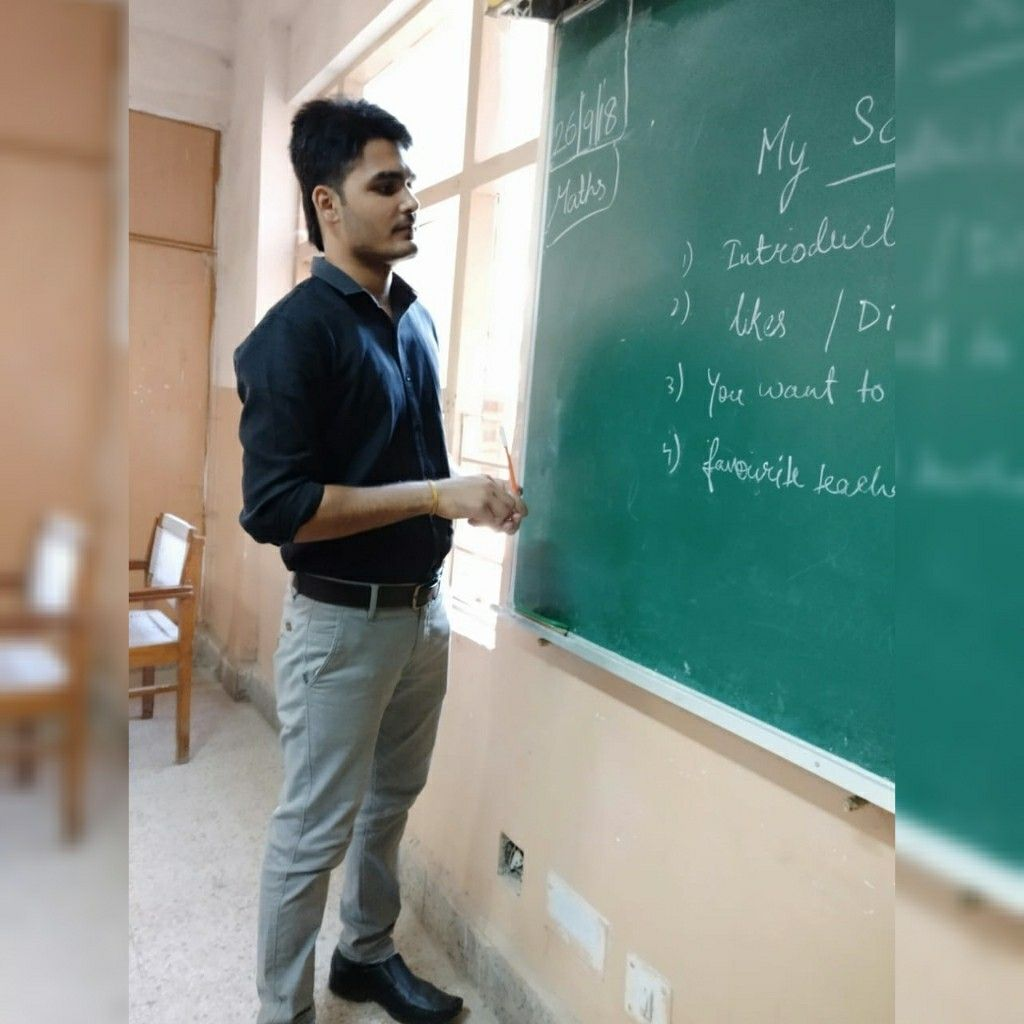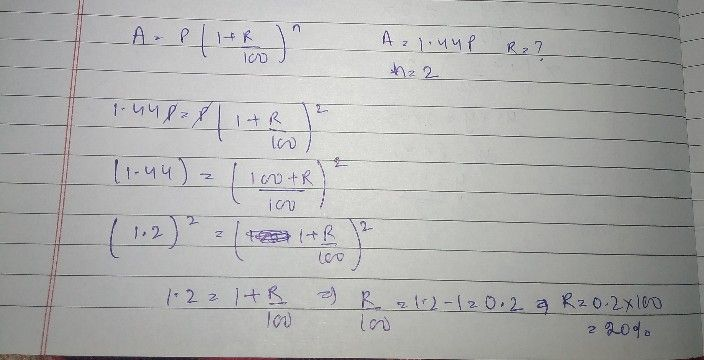Symbol
ProblemSuccess Guaranteed Classes Near Saraswati School, CRPF GO $2-69-5+5454$ Kalu $k1$ Dhani, $2kM$ from Bus Stand, $A$ $\bar{I2}$ of $202x$ compounded annually becomes $144$ times of $11$ $dtin2y$ years, then the rate of irderest tet anpum i $1\right)$ $256$ $\left(2\right)$ $226$ $\left(3\right)$ $21%$ $\left(4\right)$ $2\left(/4$ If the amount is $3\dfrac {3} {8}$ times the sum after 3 years at $8$ interest compounded
10th-13th grade
Other
SolutionQanda teacher - gaurav sirhere is your answer
i hope it will help you
please rate and review